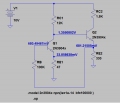# Circuit For DC Analysis

#### aac044210

Joined Nov 19, 2019
71
Normally, I would be able to perform a DC analysis of a circuit but this
one has me baffled, mainly due to the 100KΩ resistor. I would really
appreciate some help.

Thanks

#### ericgibbs

Joined Jan 29, 2010
9,117
hi aac.
Please post your asc file so that we can run the sim.
E

#### aac044210

Joined Nov 19, 2019
71
hi aac.
Please post your asc file so that we can run the sim.
E
Hi E:
Here is asc file. I guess I should have specified that I want to analyze the circuit
using loop analysis.

#### Attachments

• 1.2 KB Views: 13
Last edited:

#### Bordodynov

Joined May 20, 2015
2,455
See

#### aac044210

Joined Nov 19, 2019
71

#### ericgibbs

Joined Jan 29, 2010
9,117
hi aac.
Is this the result you are looking for.?
E

Update:
I see that @Bordodynov has got the result.#### aac044210

Joined Nov 19, 2019
71
hi aac.
Is this the result you are looking for.?
E

Update:
I see that @Bordodynov has got the result.I was hoping to figure out how to use loops and kirchoff and calculate the voltages and
currents that way. Since there is no bias voltage divider, I don't know how to proceed.

aac

#### Jony130

Joined Feb 17, 2009
5,092
I was hoping to figure out how to use loops and kirchoff and calculate the voltages and
currents that way. Since there is no bias voltage divider, I don't know how to proceed.
https://electronics.stackexchange.com/questions/305037/quiescent-point-of-two-transistors-bjts/305144#305144
And for your circuit, if you assumed β1 = β2 = ∞ we have:

Ve2 = Re2*Ic2 = Vbe1+Ic1*Re1 (1)
Vcc = Ic2*Re2 + Vbe2 + Ic1*Rc1 (2)

So we can solve for Ic1:

Ic1 = (Vcc - 2Vbe)/(Re1 + Rc1) = (10V - 1.2V)/(47Ω + 12kΩ) = 730μA

and from there we can find Ic2

Ic2 = Ve2/Re2

Ve2 = Vbe1 + Ic1*Re1 = 0.6V + 730μA*47Ω = 0.634V
and Ic2 = 1.92mA

Now we could treat this as our first iteration and in the next iteration, we could include the finite beta value.

Last edited:

#### Ylli

Joined Nov 13, 2015
851
I'm sure it can be done with the loop equations, but I would do it iteratively. You are given the DC gain of the transistors, but you would also have to know the Vbe's.

Start with the assumption that the collector of Q1 is at 1.000 volts and crunch the numbers. It will lead you to a new Q1 Vc. Crunch them again, and again, and again, until you get the precision you need.

#### aac044210

Joined Nov 19, 2019
71
https://electronics.stackexchange.com/questions/305037/quiescent-point-of-two-transistors-bjts/305144#305144
And for your circuit, if you assumed β1 = β2 = ∞ we have:

Ve2 = Re2*Ic2 = Vbe1+Ic1*Re1 (1)
Vcc = Ic2*Re2 + Vbe2 + Ic1*Rc1 (2)

So we can solve for Ic1:

Ic1 = (Vcc - 2Vbe)/(Re1 + Rc1) = (10V - 1.2V)/(47Ω + 12kΩ) = 730μA

and from there we can find Ic2

Ic2 = Ve2/Re2

Ve2 = Vbe1 + Ic1*Re1 = 0.6V + 730μA*47Ω = 0.634V
and Ic2 = 1.92mA

Now we could treat this as our first iteration and in the next iteration, we could include the finite beta value.
Ok. Ic1 = 730μA vs 692μA per LTS
Ic2 = 1.92mA vs 2.74mA per LTS
Ve2 = 0.634V vs 0.907 per LTS

There is quite a large variance.

#### Jony130

Joined Feb 17, 2009
5,092
Ok. Ic1 = 730μA vs 692μA per LTS
Ic2 = 1.92mA vs 2.74mA per LTS
Ve2 = 0.634V vs 0.907 per LTS

There is quite a large variance.
What have you expected? By assuming β = ∞ (Ib1 = Ib2 = 0A) I completely ignore the voltage drop across the RB resistor, therefore if we include the base current influence the Ve2 will be:

Ve2 = Ie1*Re1 + Vbe1+Rb*Ib1 = (Ie2 - Ib1)*Re2#### Attachments

• 1.3 KB Views: 2
Last edited:

#### aac044210

Joined Nov 19, 2019
71
What have you expected? By assuming β = ∞ (Ib1 = Ib2 = 0A) I completely ignore the voltage drop across the RB resistor, therefore if we include the base current influence the Ve2 will be:

Ve2 = Ie1*Re1 + Vbe1+Rb*Ib1 = (Ie2 - Ib1)*Re2